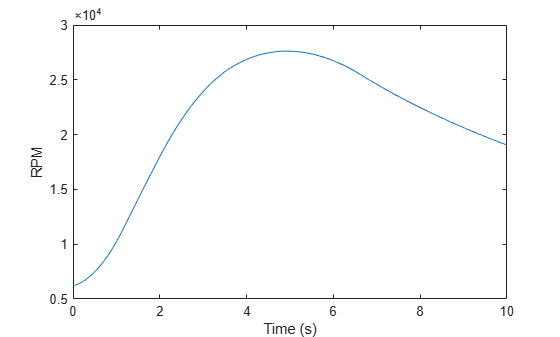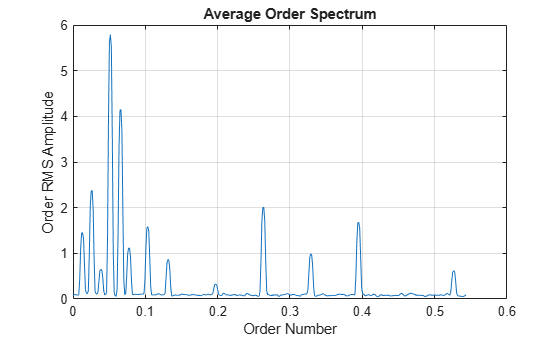# orderspectrum

Average spectrum versus order for vibration signal

## Syntax

``spec = orderspectrum(x,fs,rpm)``
``````[spec,order] = orderspectrum(x,fs,rpm)``````
``````[spec,order] = orderspectrum(map,order)``````
``````[spec,order] = orderspectrum(map,order,'Amplitude',amp)``````
``orderspectrum(___)``

## Description

example

````spec = orderspectrum(x,fs,rpm)` computes an average order-magnitude spectrum vector, `spec`, for an input signal, `x`, sampled at a rate of `fs` Hz. To compute the spectrum, `orderspectrum` windows a constant-phase, resampled version of `x` with a flat top window.```
``````[spec,order] = orderspectrum(x,fs,rpm)``` also returns a vector of the orders corresponding to each average spectrum value.```

example

``````[spec,order] = orderspectrum(map,order)``` computes an average order-magnitude spectrum vector starting from an order-RPM map and a vector of orders. Use `rpmordermap` to compute `map` and `order`. `map` must be linearly scaled. The returned amplitudes are the same as in `map`. The returned spectrum is scaled linearly.```
``````[spec,order] = orderspectrum(map,order,'Amplitude',amp)``` specifies the type of amplitude to consider when computing an average order-magnitude spectrum starting from an order-RPM map.```
````orderspectrum(___)` with no output arguments plots the RMS amplitude of the order spectrum, scaled linearly, on the current figure.```

## Examples

collapse all

Create a simulated signal sampled at 600 Hz for 5 seconds. The system that is being tested increases its rotational speed from 10 to 40 revolutions per second during the observation period.

```fs = 600; t1 = 5; t = 0:1/fs:t1; f0 = 10; f1 = 40; rpm = 60*linspace(f0,f1,length(t));```

The signal consists of four harmonically related chirps with orders 1, 0.5, 4, and 6. The order-4 chirp has twice the amplitude of the others. To generate the chirps, use the trapezoidal rule to express the phase as the integral of the rotational speed.

```o1 = 1; o2 = 0.5; o3 = 4; o4 = 6; ph = 2*pi*cumtrapz(rpm/60)/fs; x = [1 1 2 1]*cos([o1 o2 o3 o4]'*ph);```

Visualize the order-RPM map of the signal.

`rpmordermap(x,fs,rpm)`Visualize the average order spectrum of the signal. The peaks of the spectrum correspond to the ridges seen in the order-RPM map.

`orderspectrum(x,fs,rpm)`Analyze simulated data from an accelerometer placed in the cockpit of a helicopter.

Load the helicopter data. The vibrational measurements, `vib`, are sampled at a rate of 500 Hz for 10 seconds. The data has a linear trend. Remove the trend to prevent it from degrading the quality of the order estimation.

```load('helidata.mat') vib = detrend(vib);```

Plot the nonlinear RPM profile. The rotor runs up until it reaches a maximum rotational speed of about 27,600 revolutions per minute and then coasts down.

```plot(t,rpm) xlabel('Time (s)') ylabel('RPM')```Compute the average order spectrum of the signal. Use the default order resolution.

`orderspectrum(vib,fs,rpm)`Use `rpmordermap` to repeat the computation with a finer order resolution. The lower orders are resolved more clearly.

```[map,order] = rpmordermap(vib,fs,rpm,0.005); orderspectrum(map,order)```Compute the power level for each estimated order. Display the result in decibels.

```[map,order] = rpmordermap(vib,fs,rpm,0.005,'Amplitude','power'); spec = orderspectrum(map,order); plot(order,pow2db(spec)) xlabel('Order Number') ylabel('Order Power Amplitude (dB)') grid on```## Input Arguments

collapse all

Input signal, specified as a row or column vector.

Example: `cos(pi/4*(0:159))+randn(1,160)` specifies a sinusoid embedded in white Gaussian noise.

Data Types: `double` | `single`

Sample rate, specified as a positive scalar expressed in Hz.

Data Types: `double` | `single`

Rotational speeds, specified as a vector of positive values expressed in revolutions per minute. `rpm` must have the same length as `x`.

• If you have a tachometer pulse signal, use `tachorpm` to extract `rpm` directly.

• If you do not have a tachometer pulse signal, use `rpmtrack` to extract `rpm` from a vibration signal.

Example: `100:10:3000` specifies that a system rotates initially at 100 revolutions per minute and runs up to 3000 revolutions per minute in increments of 10.

Data Types: `double` | `single`

Order-RPM map, specified as a matrix. Use `rpmordermap` to compute order-RPM maps.

Data Types: `double` | `single`

Orders in order-RPM map syntax, specified as a vector. The length of `order` must equal the number of rows in `map`.

Data Types: `double` | `single`

Order-RPM map amplitudes, specified as one of `'rms'`, `'peak'`, or `'power'`.

• `'rms'` — Assumes that the order-RPM map uses the root-mean-square amplitude for each estimated order.

• `'peak'` — Assumes that the order-RPM map uses the peak amplitude for each estimated order.

• `'power'` — Assumes that the order-RPM map uses the power level for each estimated order.

## Output Arguments

collapse all

Average order-magnitude spectrum, returned as a vector of root-mean-square (RMS) amplitudes in linear scale. If you use `map` and `order` as input arguments, and set `'Amplitude'` to `'power'` when using `rpmordermap` to compute `map`, then `orderspectrum` returns `spec` in power units.

Output orders, returned as a real vector.

 Brandt, Anders. Noise and Vibration Analysis: Signal Analysis and Experimental Procedures. Chichester, UK: John Wiley & Sons, 2011.

 Vold, Håvard, and Jan Leuridan. “High Resolution Order Tracking at Extreme Slew Rates Using Kalman Tracking Filters.” Shock and Vibration. Vol. 2, 1995, pp. 507–515.